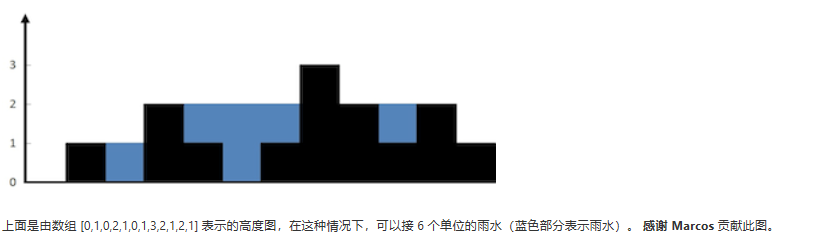# 27.LeetCode刷题之路42题接雨水

2020年6月11日14:58:43评论78阅读模式

### 题目链接

https://leetcode-cn.com/problems/trapping-rain-water/文章源自随机的未知-https://sjdwz.com/11134.html

### 题目内容### 思路

right_height_array表示当前柱子后面的所有柱子的最高值（不包括当前柱子）；

（其实可以在比较left_height_array[i]right_height_array[i] 大小关系时就可以进行累加存水量了。）文章源自随机的未知-https://sjdwz.com/11134.html

### 案例分析

height : [0,1,0,2,1,0,1,3,2,1,2,1]

0，0，1，1，2，2，2，2，3，3，3，3

3，3，3，3，3，3，3，2，2，2，1，0；
height数组为：
0，1，0，2，1，0，1，3，2，1，2，1
index=0时，left_height_array = 0,right_height_array = 0不能放水，存水量为0；
index=1时，left_height_array = 0,right_height_array = 3不能放水，两者最小为0，存水量为0；
index=2时，left_height_array = 1,right_height_array = 3不能放水，两者最小为2，而height[i]为0，存水量为两者最小值 - height[i] = 1；

### 代码

``````class Solution {
public int trap(int[] height) {
int result = 0;
if(height.length == 0) return result;
int[] left_height_array = new int[height.length];
int[] right_height_array = new int[height.length];
int left_max = 0;
int right_max = 0;
left_height_array = 0;
right_height_array = 0;
for(int i = 1;i<height.length;i++){
if(height[i-1]>left_max){
left_max = height[i-1];
}
left_height_array[i] = left_max;
}

for(int i = height.length -2;i>=0;i--){
if(height[i+1]>right_max){
right_max = height[i+1];
}
right_height_array[i] = right_max;
}
for(int i=0;i<height.length;i++){
if(left_height_array[i] == right_height_array[i]){
if(left_height_array[i] > height[i])
result+=left_height_array[i]-height[i];

}else if(left_height_array[i] < right_height_array[i]){
if(left_height_array[i] > height[i])
result+=left_height_array[i]-height[i];
}else{
if(right_height_array[i]>height[i])
result += right_height_array[i] - height[i];
}
}
return result;
}
}
``````

### 欢迎关注文章源自随机的未知-https://sjdwz.com/11134.html

• 本文由 发表于 2020年6月11日14:58:43
• 转载请注明：来源：随机的未知 本文链接https://sjdwz.com/11134.html